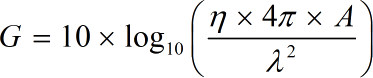Request a Tool

Antenna Gain Calculator

The antenna gain in a given direction is the difference between the energy radiated in that direction and the energy radiated in the same direction by an isotropic antenna powered with the same input power.

Array Factor
0

Formula• π = a constant value 3.1415
• η = Efficiency
• λ = Wave Length
• A = Physical Aperture Area

Defination / Uses

The combination of the antenna's directivity and electrical efficiency is referred to as antenna gain. Efficiency, Wave Length, and Physical Aperture Area are used to calculate antenna gain. To put it another way, antenna gain is the ratio of the power required at the input of a loss-free reference antenna to the power delivered to the input of the given antenna to create.

The gain of a transmitting antenna indicates how successfully it converts input power into radio waves that are directed in a certain direction. The gain of a receiving antenna indicates how successfully it transforms radio waves from a particular direction into electrical power.

How antenna gain calculator is helpfull

The sign 'dB' stands for antenna gain. The 'dB' stands for decibel, which is a measurement unit for loss or gain. An antenna's length is equal to the square root of its gain for a given frequency and radiation resistance in antenna gain. Gain Antenna Calculator helps to measure accurate frequency of antennas. By using Efficiency, Wave Length and Aperture Area as in the above given formula, we can calculate the Antenna frequency. Calculator saves your time as compared to calculate manually. You just have to add the values in fields and get perfect answer in less than a second.